Processing ......FreeComputerBooks.com Links to Free Computer, Mathematics, Technical Books all over the World

Lectures on Probability Theory and Mathematical Statistics
Top Free Machine Learning Books 🌠 - 100% Free or Open Source!
• Title: Lectures on Probability Theory and Mathematical Statistics
• Author(s) Marco Taboga
• Publisher: Statlect.com
• Hardcover/Paperback: N/A
• eBook: HTML
• Language: English
• ISBN-10/ASIN: N/A
• ISBN-13: N/A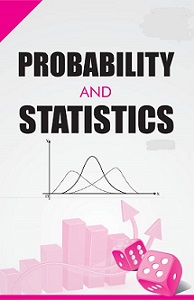Book Description

This is a collection of lectures on probability theory and mathematical statistics written by Marco Taboga, a professional financial economist with a passion for mathematics. It is offered as a free service to the mathematical community and provides an accessible introduction to topics that are not usually found in elementary textbooks. It collects results and proofs, especially on probability distributions, that are hard to find in standard references and are scattered here and there in more specialistic books.

These lectures have been in the recommended reading lists of statistics classes in several universities, including Dartmouth College, Michigan State University, University of North Carolina - Chapel Hill, Stanford University, University of Texas - Austin, Yale University, Washington University, University of Wisconsin, as well as in many other universities both in the US and in the rest of the world.

• Marco Taboga is a pricipal economist at the Bank of Italy, where he has led teams of PhDs in mathematics and economics. He holds a PhD in applied mathematics and a Master in finance from the London School of Economics and Political Science. He has taught mathematics and statistics in university courses. He has published several articles in top scholarly journals, including Mathematical Finance, The Journal of Money Credit and Banking and International Finance.
Reviews, Ratings, and Recommendations: Related Book Categories: Read and Download Links:Similar Books:
•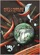Mostly Harmless Statistics (Rachel L. Webb)

This text is for an introductory level probability and statistics course with an intermediate algebra prerequisite. The focus of the text follows the American Statistical Association's Guidelines for Assessment and Instruction in Statistics Education (GAISE).

•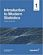Introduction to Modern Statistics (Mine Çetinkaya-Rundel, et al.)

This book puts a heavy emphasis on exploratory data analysis and provides a thorough discussion of simulation-based inference using randomization and bootstrapping, followed by a presentation of the related Central Limit Theorem based approaches.

•Foundations in Statistical Reasoning (Pete Kaslik)

This book is designed for students taking an introductory statistics class. The emphasis throughout the entire book is on how to make decisions with only partial evidence. It focuses on the thought process.

•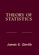Theory of Statistics (James E. Gentle)

This book is directed toward students for whom mathematical statistics is or will become an important part of their lives. It covers classical likelihood, Bayesian, and permutation inference; an introduction to basic asymptotic distribution theory; and modern topics.

•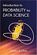Introduction to Probability for Data Science (Stanley Chan)

This book is an introductory textbook in undergraduate probability in the context of data science to emphasize the inseparability between data (computing) and probability (theory) in our time, with examples in both MATLAB and Python.

•Introduction to Probability, Statistics, and Random Processes

This book introduces students to probability, statistics, and stochastic processes. It provides a clear and intuitive approach to these topics while maintaining mathematical accuracy. The book contains a large number of solved exercises.

•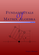Fundamentals of Matrix Algebra (Gregory Hartman)

A college (or advanced high school) level text dealing with the basic principles of matrix and linear algebra. It covers solving systems of linear equations, matrix arithmetic, the determinant, eigenvalues, and linear transformations.

•Matrix Algebra (Marco Taboga)

This is a course in matrix algebra, with a focus on concepts that are often used in probability and statistics. Matrix algebra is one of the most important areas of mathematics for data analysis and for statistical theory.

Book Categories
 :All CategoriesTop Free BooksRecent BooksMiscellaneous BooksComputer EngineeringComputer LanguagesComputer ScienceData Science/DatabasesJava and Java EE (J2EE)Linux and UnixMathematicsMicrosoft and .NETMobile ComputingNetworking and CommunicationsSoftware EngineeringSpecial TopicsWeb Programming
Other Categories